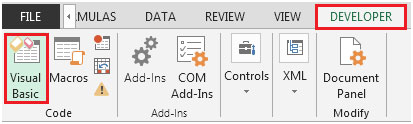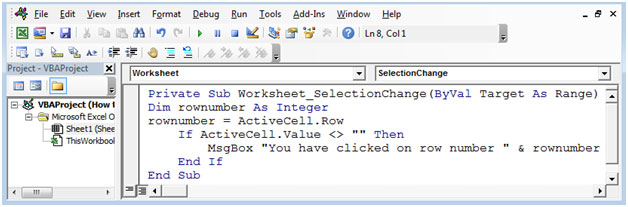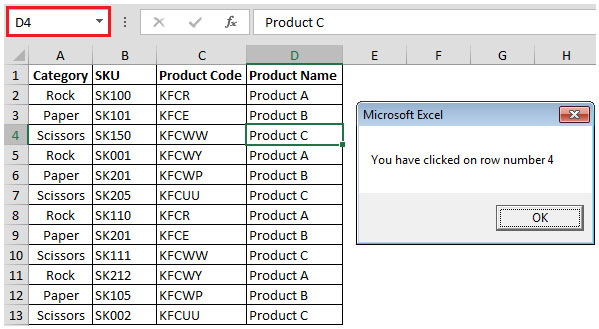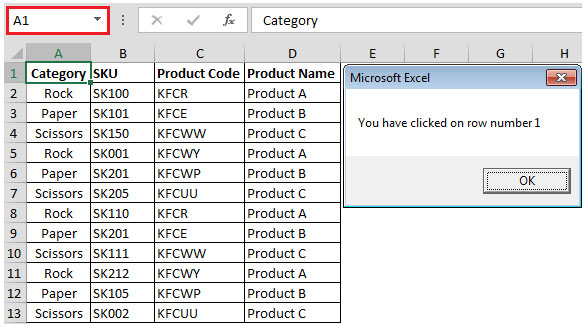# How To Get Row Number By A Single Click Using VBA In Microsoft Excel 2010

In this article, you will learn how to get row number by a single click.

Click on Developer tab
From Code group, select Visual BasicEnter the following code in the current worksheet (sheet1 in our example)

Private Sub Worksheet_SelectionChange(ByVal Target As Range)

Dim rownumber As Integer

rownumber = ActiveCell.Row

If ActiveCell.Value <> "" Then

MsgBox "You have clicked on row number " & rownumber

End If

End SubThe SelectionChange event will get activated every time the user selects any cell & it will give us the row number of the selected cell. If active cell is empty then, the code will not run.In above snapshot, you can see the formula bar contains cell D4 & hence the row number is 4.

If we select cell A1, and then we get the row number as 1. Refer below shown snapshotIn this way, you can get the row number of the selected cell, using VBA code.

1.